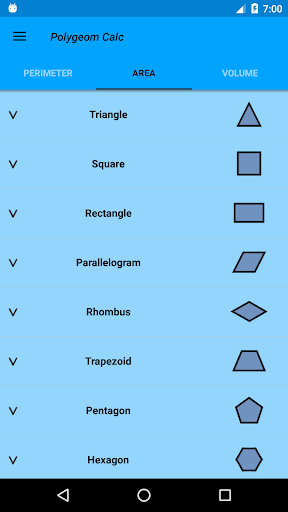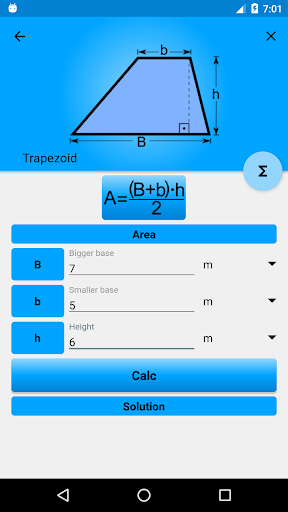Sensational application, easy, intuitive, practical and very useful for calculating formulas of plane and spatial geometry. Calculating the angle, perimeter, area, and volume of geometric figures has never been easier.

==> Geometric figures:

★ Complementary Angle;
★ Supplementary Angle;
★ Triangle;
★ Triangle Equilateral;
★ Equilateral Triangle Inscribed in Circumference;
★ Internal Angles of the Triangle;
★ External Angles of the Triangle;
★ Pythagorean Theorem;
★ Rectangle;
★ Square;
★ Square Inscribed in Circumference;
★ Parallelogram;
★ Rhombus;
★ Trapezium;
★ Regular Pentagon;
★ Regular Hexagon;
★ Regular Hexagon Inscribed in Circumference;
★ Ellipse;
★ Circle;
★ Circular Crown;
★ Circular Sector;
★ Circular Crown Sector;
★ Cube;
★ Parallelepiped;
★ Square Base Pyramid;
★ Truncated Pyramid with Parallel Bases;
★ Cylinder;
★ Hollow Cylinder;
★ Cone;
★ Cone Truncated with Parallel Bases;
★ Sphere;
★ Hollow sphere;
★ Ellipsoid;
★ Circular Torus.

★ Integrated metric unit converter.

Did not you find the formula you wanted? Keep Calm!
Leave your opinion in the comments and indicate the formula you did not find.

Help the developer with a good evaluation!

Thanks for listening!
# V1.1.0
– New formulas.
– Improvements.
– Translation into other languages.
– More updates and improvements soon!